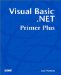# Review Questions

 1: When do you use a While End While loop? A1: While End While loops are normally used when the number of iterations that must be made through the loop is unknown when the loop is entered. This is unlike most For loops, where the number of passes to be made is often known before the loop begins execution. 2: Convert the following code to a While loop: ` For i = 20 to 0 Step -1 Sum += cint(i) Next i ` A2: One way is ` Dim i, sum As Integer sum = 0 i = 20 While i > 0 sum = sum + i i = i - 1 End While ` Notice that the three conditions for a well-behaved loop are still present. 3: Use a While loop to find the factorial of 10. (Recall that a factorial is the product of all integer values from 1 to the number being factored . For example, 5 factorial is 5 * 4 * 3 * 2 * 1 = 120.) A3: Again, there are various ways that this could be written, but one simple one is ` Dim num, factorial As Double num = 9 factorial = num + 1 While num > 0 factorial *= num num -= 1 End While ` 4: What is the primary difference between a Do While loop and a Do Until loop. A4: A Do While loop executes as long as the test expression evaluates to logic True. A Do Until loop executes as long as the expression is logic False. 5: Assume that a huge text buffer named buff holds thousands of words from a text file. You want to count the frequency of each letter of the alphabet found in the text file. Uppercase and lowercase letters are treated the same. At the end of the program, you want to show the counts for each letter. How would you write the code? A5: Try: ` Dim i As Integer, length As Integer, index As Integer Dim buff As String, Counts(26) As Integer buff = "aeeeeeeemmmmmmmg" For i = 1 To Len(buff) index = Asc(UCase(Mid\$(buff, i, 1))) - Asc("A") Counts(index) = Counts(index) + 1 Next i For i = 0 To 25 If Counts(i) <> 0 Then Debug.Print Chr\$(i + 65); Counts(i) End If Next i ` 6: Suppose that you're a cryptography expert. You're sent a file that you know contains a hidden message. You also know that the letters in the message are N letters apart, but you've forgotten what N is. Write a program that looks for the correct N to decipher the message. You can use the following short piece of text to test your program. When N is correct, a message should appear. ` "vBiGl;iowQs fe BhrloZdpiM ylBFwe/']rqadsbaw" ` A6: One way might be ` Dim bump, i, length As Integer Dim buff As String buff = "vBiGl;iowQs fe BhrloZdpiM ylBFwe/']rqadsbaw" length = buff.Length bump = 0 Do While True bump += 1 console.system.write ("bump = "; bump; " ") For i = 1 To length If i + bump > length Then Exit For End If console.system.write (Mid\$(buff, i + bump, 1)) i = i + bump Next i console.system.writeline("") If bump > length / 2 Then Exit Do End If Loop ` In the program, bump is the factor that sets the spaces between characters . The For loop uses bump to march along the string. (You could use a For loop with a Step argument is you chose.) The two If statements are used to prevent unnecessary passes through the loop. (How does it do that?)Visual Basic .NET Primer Plus
ISBN: 0672324857
EAN: 2147483647
Year: 2003
Pages: 238
Authors: Jack Purdum

Similar book on Amazon